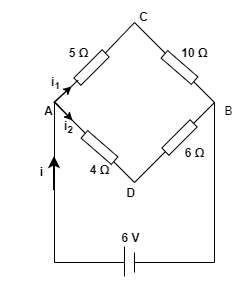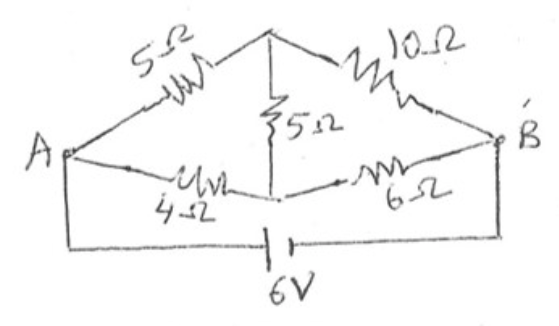# Problem: What is the current through the battery in the circuit shown below? What is the equivalent resistance between A and B for the network of resistors?

###### FREE Expert Solution

(A)

The voltage at A, B, C, and D are VA, VB, VC, and VD. The current through AC is i1 and the current through AD is i2. The total current is i.Applying the nodal analysis law to VC:

(VC - VA)/5Ω + (VC - VD)/5Ω + (VC - VB)/10Ω = 0

(VC - 6)/5Ω + (VC - VD)/5Ω + VC/10Ω = 0

(1/5 + 1/5 + 1/10)VC - (1/5)VD = 6/5

88% (326 ratings)###### Problem Details

What is the current through the battery in the circuit shown below? What is the equivalent resistance between A and B for the network of resistors?